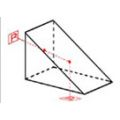Product Description

A Right-Angle Prism is used as a mirror to deviate light through 90 degree, and also as a retro reflector to deflect light through 180 degree by total internal reflection.
………………………………………………………………….
BK7 Precision Right-Angle Prism

Specification:

Material: BK7 glass
Dimension Tolerance: +0.0, -0.2 mm
Clear Aperture: >80%
Angle Tolerance: see the table
Flatness: Lambda/2 at 632.8 nm
Surface Quality: 60-40 scratch and dig
Protective Bevel3 min. deviation
Size(mm) Part No.
A=B=C=3.2 RAP0001
A=B=C=5.0 RAP0101
A=B=C=10.0 RAP0201
A=B=C=12.7 RAP0301
A=B=C=15.0 RAP0401
A=B=C=20.0 RAP0501
A=B=C=25.4 RAP0601
A=B=C=30.0 RAP0901
A=B=C=40.0 RAP0701
A=B=C=50.8 RAP0801